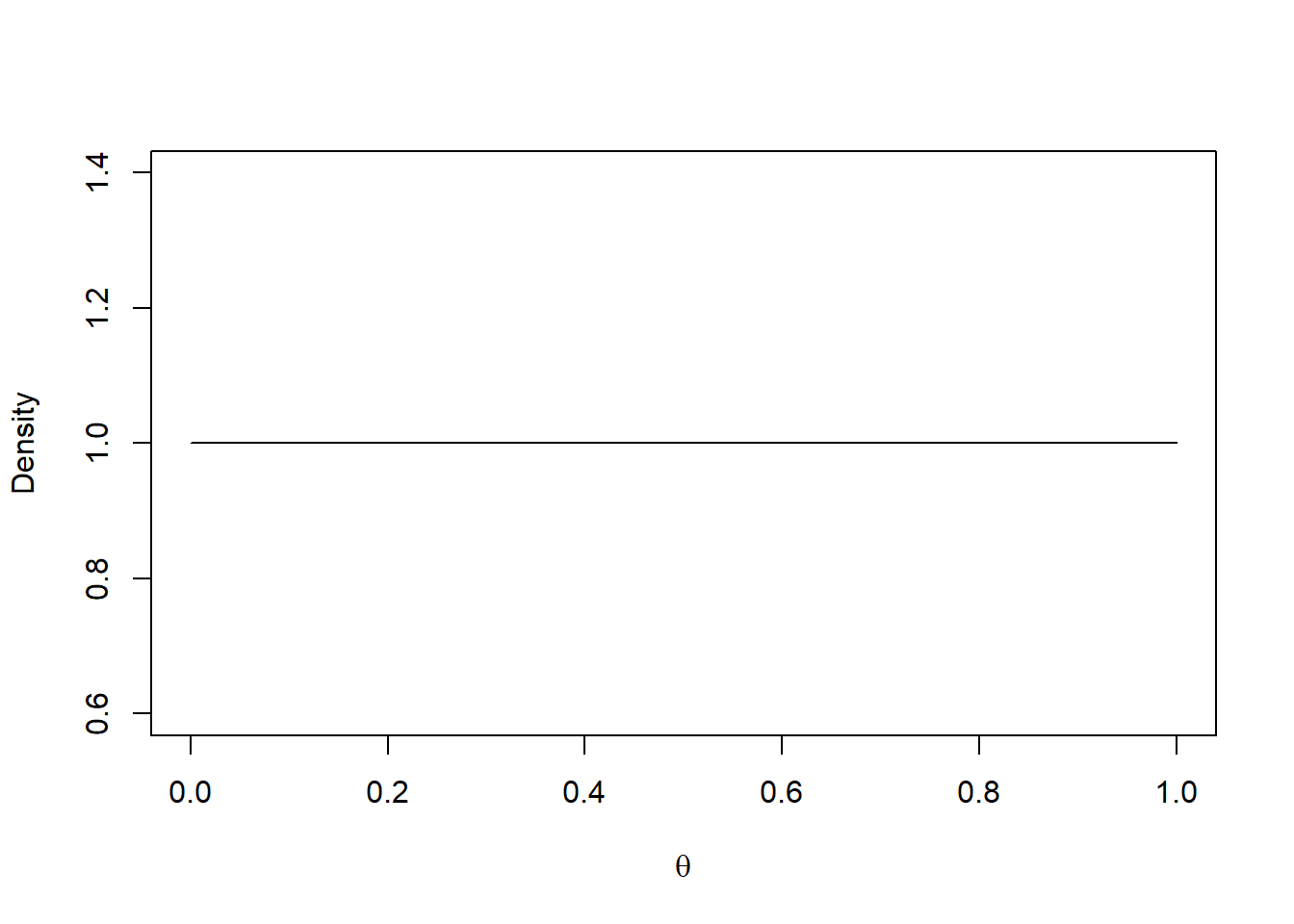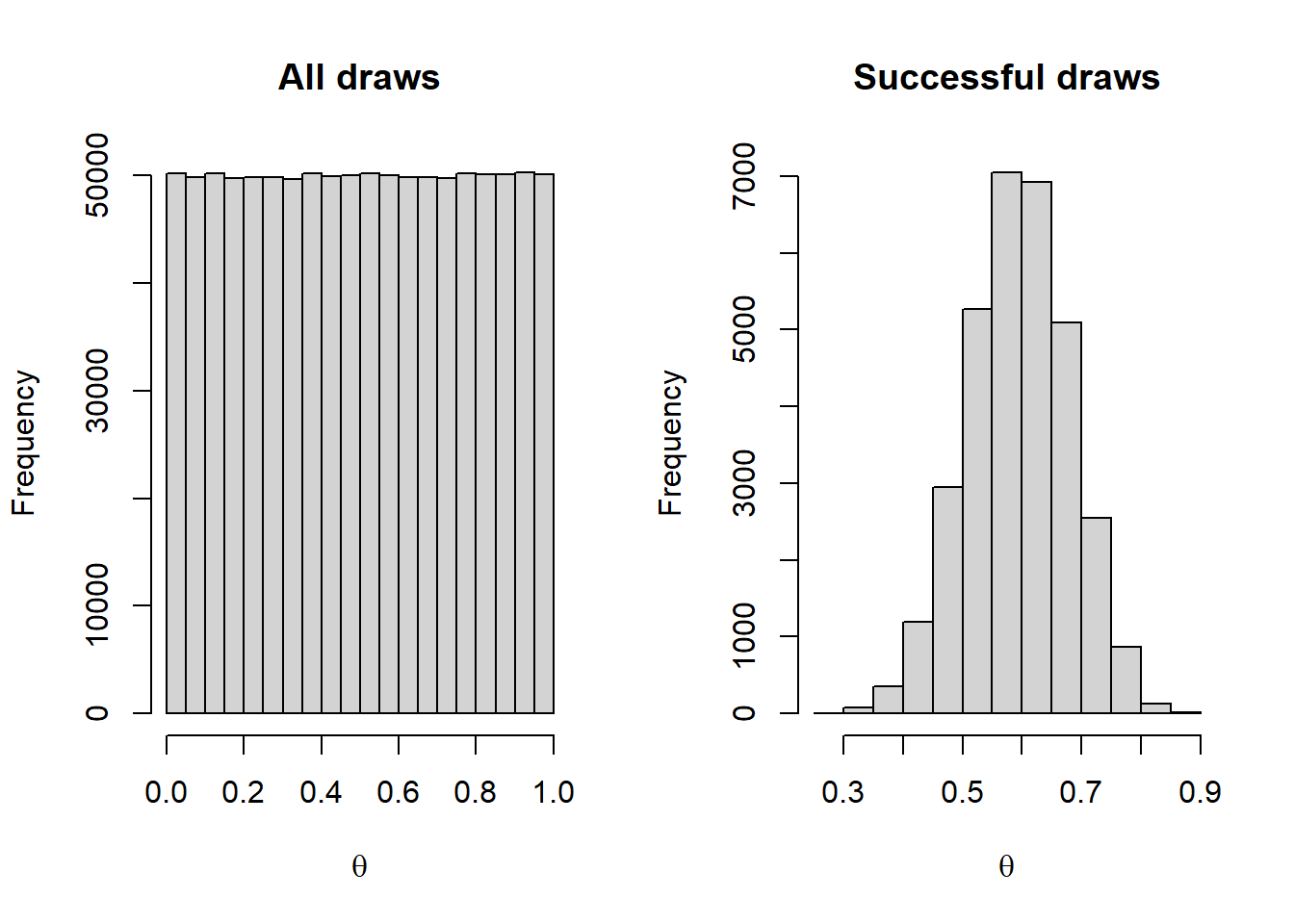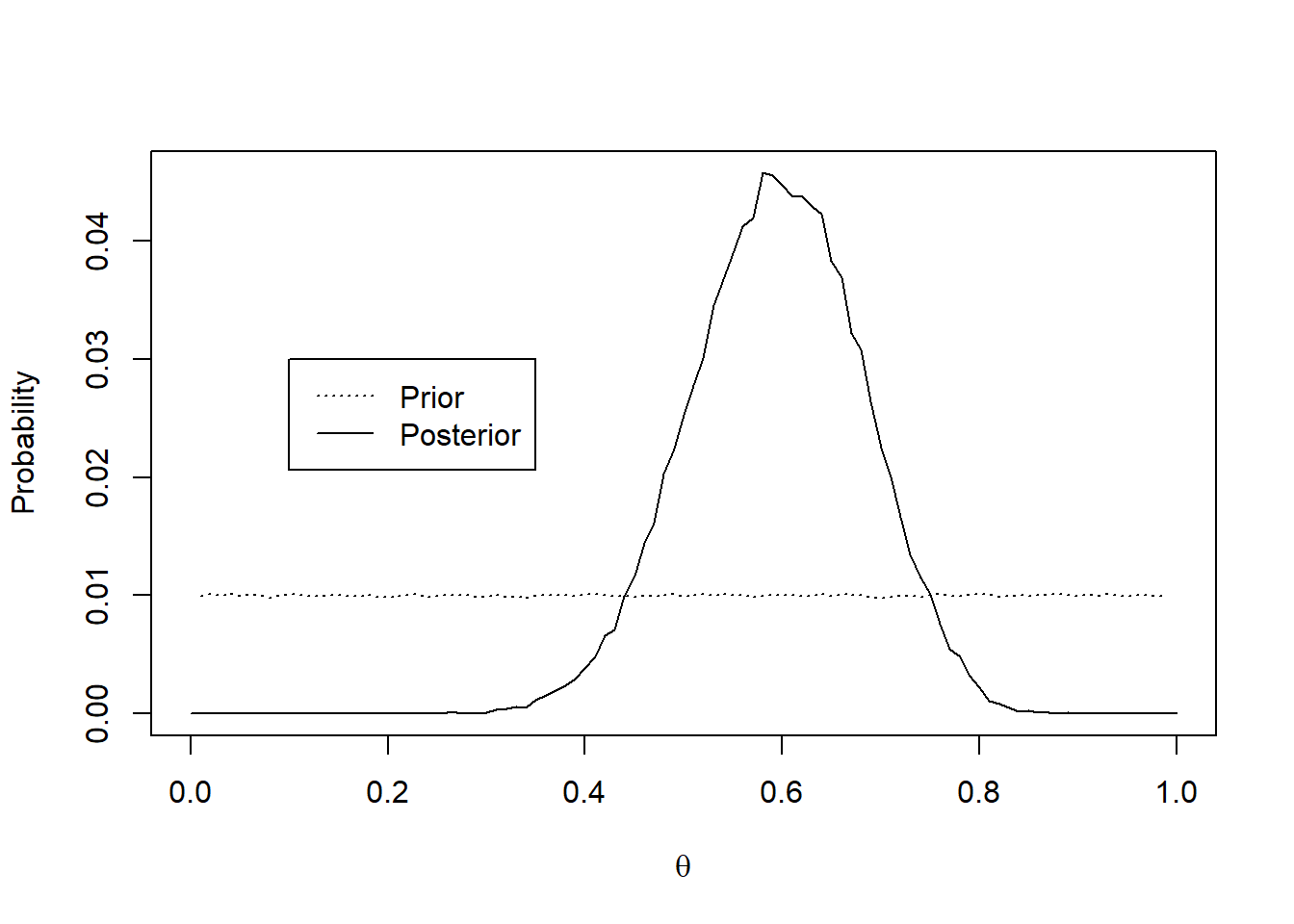The Bayes theorem states that the posterior probability of a model, given the data, is proportional to the joint probability of the data given the model and the prior probability of the model, normalized over the probability of the data itself More succinctly: $P(\theta|y) \propto \frac{P(y|\theta)P(\theta)}{P(y)}$

where:

• $$P(\theta|y)$$ : Probability of the model given the data
• $$P(y|\theta)$$ : Probability of the data given the model
• $$P(\theta)$$ : Prior probability of the model
• $$P(y)$$ : Prior probability of the data

In order to estimate the probability of a model, we need three components: some data, a generative model, and a prior belief.

Let’s start with some data. Suppose we want to evaluate the efficacy of a vaccine in people. The outcome of our intervention is binary (either the vaccine prevented disease over a 1 year period, or it didn’t).

Here’s the data:

vacdat <- c(rep(1, 18), rep(0, 12))

Simple enough. Our sample is composed by 30 subjects. The vaccine worked for 18 of them, but it didn’t for the remaining 12 subjects.

Let’s go back to the initial requirements for Bayesian model estimation. First, we have our data. How does this data distribute? Here, we want to be explicit in our assumptions. We’ll say that our data follows the binomial distribution with parameter $$\theta$$, which represents the probability that the vaccine prevents the disease (1, a success). $vacdat \sim Binomial(\theta)$ This amounts to specifying a generative model. We believe that this model, under a specific parameterization, created our data.

But how does $$\theta$$ itself distribute? We know theta ought to be bound between 0 and 1, so we could use the Beta distribution. But, the Beta distribution itself takes two shape parameters ??dbeta. What should those parameters be? Here’s were our prior beliefs come into play.

Let’s be as naive as possible, and consider that the efficacy of our vaccine can span the whole range of efficacy. It can go from completely failing to prevent the disease (i.e., $$\theta = 0$$) to completely preventing it (i.e., $$\theta = 1$$). Moreover, any of these possibilities is equally likely. Under these beliefs, the best prior distribution for $$\theta$$ is: $\theta \sim Beta(1, 1)$ Let’s see what this distribution looks like:

thetas <- seq(0, 1, .01)
plot(thetas, dbeta(thetas, 1, 1), type = 'l', xlab = expression(theta), ylab = 'Density')Now we are ready to tackle this problem. Simulation is often the best tool to get an understanding of this, so let’s simulate some data. Here is the algorithm we are going to use:

For N draws:

1. Sample $$\theta$$ from $$Beta(1,1)$$
2. Generate n samples $$s$$ from $$Binomial(\theta)$$

With this in hand, let’s perform 100K draws.

ndraws <- 1e6
thetas <- rbeta(ndraws, 1, 1)
samples <- rbinom(ndraws, 30, prob = thetas)
draws <- data.frame(theta = thetas, successes = samples)

Now, we can visualize the distribution of $$\theta$$s we sampled (which should be representative of our prior), and another distribution containing the $$\theta$$s that yielded an equal number of successes as we have in the real data.

{op <- par(mfrow = c(1, 2))
hist(draws$theta, xlab = expression(theta), main = 'All draws') hist(draws$theta[draws$successes == sum(vacdat)], xlab = expression(theta), main = 'Successful draws') par(op)}We can clearly see not all $$\theta$$s produce the data we observed with the same probability. Low and high $$\theta$$s are very unlikely to produce our data, but $$\theta$$s that approach the proportion of successes we observed do so with a higher probability. More importantly, the distribution of successful draws will be proportional to the posterior probability distribution of $$\theta$$. To precisely calculate this posterior distribution, we have to normalize the joint probability $$P(y|\theta)P(\theta)$$ by the probability of our data $$P(y)$$. In most tutorials (and in practice), this quantity is often ignored (as it involves nasty integrals or expensive approximations). However, we will go the extra mile. One way to approximate $$P(y)$$ is: $P(y) = \sum P(y|\theta)P(\theta)$ over all individual $$\theta$$s we drew. For this case, lets perform this approximation over $$\theta$$s that are rounded to two decimal places. Although we will lose resolution, This will let us create meaningful estimations with our limited draws. After rounding $$\theta$$, we can calculate $$P(y)$$, and compute the posterior for this coarser parameter space. draws$rtheta <- round(draws$theta, digits = 2) utheta <- sort(unique(draws$rtheta))
conditionals <- sapply(utheta, function(x)
sum(draws$rtheta == x & draws$successes == sum(vacdat))/sum(draws$rtheta == x)) priors <- sapply(utheta, function(x) sum(draws$rtheta == x)/ndraws) #pardon the tails

Let’s plot the prior and posterior probabilities at the same time.

{plot(utheta, conditionals*priors/sum(conditionals*priors), type = 'l',
xlab = expression(theta), ylab = 'Probability')
lines(utheta[c(-1, -length(utheta))], priors[c(-1, -length(utheta))], lty = 'dotted')}
legend(.1, .03, legend=c("Prior", "Posterior"), lty=c("dotted", "solid"))Updating our prior beliefs after observing data is what Bayes is all about!